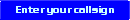Welcome to my site!JT4
Slow Mode high Signal noise ratio mode

This mode is indented for very slow and extremely weak signals at 4.375 baud

The author labled this mode as a 4-toned FSK that is why it is under FSK type of modes

FEC in JT4 uses a strong convolutional code with constraint length K=32, rate r=1/2, and a zero tail.
This choice leads to an encoded message length of (72+31) x 2 = 206 information-carrying bits.
Modulation is 4-tone frequency-shift keying (4-FSK) at 11025 / 2520 = 4.375 baud.
Each symbol carries one information bit (the most significant bit) and one synchronizing bit.
The two 32-bit polynomials used for convolutional encoding have hexadecimal values 0xf2d05351 and 0xe4613c47, and the ordering of encoded bits is scrambled by an interleaver. The pseudo-random sync vector is the following sequence (60 bits per line):

000011000110110010100000001100000000000010110110101111101000
100100111110001010001111011001000110101010101111101010110101
011100101101111000011011000111011101110010001101100100011111
10011000011000101101111010

Min. SNR is -23 db referenced to 2500hz bandwidth noise levels
TX duration is 47.1 seconds
Bandwidth is 17.5 hz
Modulation: 4-FSK

If you ran across this mode on the air and was not familair to this mode , you might think this is a birdie in your radio , due to the small bandwidth.
eQSL
checkt to see if you have
any eQSL cards!LotW
Club Log